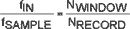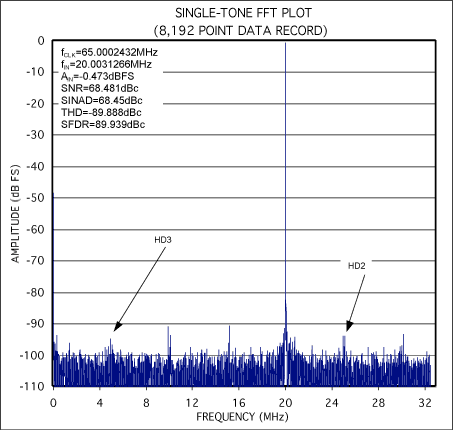# Coherent Sampling Calculator (CSC)

### Abstract

This calculator provides a quick and easy way to select sinusoidal input test tones for the test of analog-to-digital (ADC) converters. Based on the theory of coherent sampling, the calculator allows a user to chose input and sampling frequencies as well as number of integer cycles within the sampling window and the resolution (number of data point) of the FFT.

When evaluating the dynamic performance of analog-to-digital converters (ADCs) using Fast Fourier Transforms (FFTs), one must decide whether to use coherent sampling or window sampling. Maxim's application note "Coherent Sampling vs. Window Sampling" details the advantages and disadvantages of each sampling technique, but, in general, coherent sampling produces the best quality in high-resolution FFTs.

The purpose of coherent sampling is to force an integer number of input cycles within the sampling window. Figure 1 shows several examples of coherent sampling in the time domain.Figure 1a-d. Coherently sampled data contains an integer number of cycles within the sampling window. These figures show four sets of coherently sampled data. Each data set has 13 cycles within the sampling window and contains 64 data points. NWINDOW = 13, NRECORD = 64

Mathematically, coherent sampling is expressed as:where:

• fIN is the input frequency of the ADC under test.
• fSAMPLE is the sampling frequency of the ADC under test.
• NWINDOW is the integer number of cycles within the sampling window. This value must be an odd or prime number.
• NRECORD is the number of data points used to create your FFT. This value must be a power of 2.

Finding an fIN and an fSAMPLE that satisfy the above relationship is an iterative process. An Excel spreadsheet titled "Coherent Sampling Calculator" is available for download to simplify the process. Alternatively, a simplified web-based version of the Coherent Sampling Calculator is available.

The "Coherent Sampling Calculator" requires four input variables:

• fDSAMPLE is the desired sampling frequency of the ADC under test.
• fDIN is the desired input frequency of the ADC under test.
• NRECORD is the number of data points used to create your FFTs. This value must be a power of 2.
• SIGFIG is the desired number of significant figures after MHz in the coherent sampling results.

From the four variables listed above the "Coherent Sampling Calculator" will provide a coherent sampling frequency (fCSAMPLE) and input frequency (fCIN).

The Coherent Sampling Calculator includes a macro that allows the user to view the detailed intermediate steps of the calculation process. By default, the spreadsheet is set to hide these detailed calculations, however by clicking the "Show_Detailed_Calculations" button the user can get additional information such as the size of the coherent sampling window (tCWINDOW) and the number of input cycles in the coherent sampling window (NCWINDOW).

Figures 2-3 show examples of coherently sampled FFTs taken using the coherent sampling calculator and the MAX1211EVKIT.